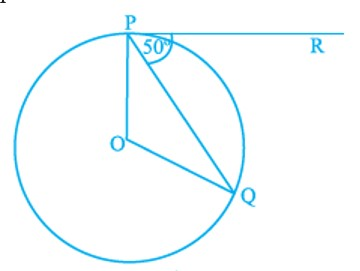#### In Figure, if O is the centre of a circle, PQ is a chord and the tangent PR at P makes an angle of 50° with PQ, then ∠POQ is equal to(A) 100°          (B) 80°              (C) 90°             (D) 75°Solution
Given :
We know that tangent is perpendicular to radius.
Hence
OPR =
OPR = OPQ + QPR
= OPQ +
OPQ =
OPQ = OQD
Hence , OPQ =
We know that the sum of interior angles of a triangle is
In
O+Q +P =
O +40+40 = 180
o =
Hence OPQ =